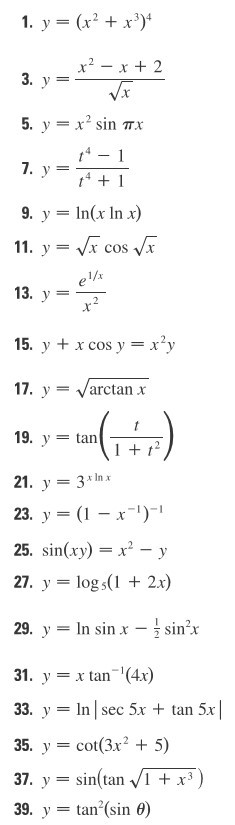1. У(x2 + x3)49. Уln(x ln x)1 + t21. У 3x1n х23, y = (1-x-1)-25. sin(xy)-x2-y27. y-logs 2x)29. y--In sin x--sin-х1. yan (4x)33. yInsec 5x + tan 5x35, y = cot(3x2 + 5)37. y=sin(tan-/I +x3

Question

Differentiation#11help_outlineImage Transcriptionclose1. У (x2 + x3)4 9. У ln(x ln x) 1 + t 21. У 3x1n х 23, y = (1-x-1)- 25. sin(xy)-x2-y 27. y-logs 2x) 29. y--In sin x--sin-х 1. yan (4x) 33. yInsec 5x + tan 5x 35, y = cot(3x2 + 5) 37. y=sin(tan-/I +x3 fullscreen
Step 1

We have

Step 2

Formula used:

Step 3

Now differentiate y with respect ...

Want to see the full answer?

See Solution

Want to see this answer and more?

Our solutions are written by experts, many with advanced degrees, and available 24/7

See Solution
Tagged in

Calculus